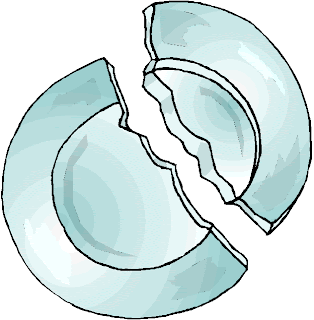## Angle Sum of Polygons in Tessellated Triangles

Tessellated triangles are not only beautiful but that they are also interesting. Tessellating them will prove the angle sum of polygons particularly parallelogram, trapezoid, and hexagon. The term angle sum means the sum of the interior angles.

Let us start with the knowledge that the angle sum of a triangle is 180 degrees. Copying a triangle with angle measures x, y, and z, and rotating it 180 degrees will give us the first two figures. The tessellated copies are shown in the next figure.Using the three figures above, we can prove the following.  » Read more

## How to Find the Center of a Circle and The Mathematics Behind

Finding the center of a circle can be quite tricky in some cases, but has a practical and important applications. For instance, an ancient broken  plate is unearthed and the archaeologist wants to find the length of its diameter, he can just find its center. Once the center is found, he can also find the length of the radius and hence can find the length of the diameter.In this post, we learn how to find the center of the circle in different ways.  » Read more

## The 3 Ancient Construction Problems

Problems about construction using compass and straightedge preoccupied mathematicians for 2000 years. The first three postulates of Euclid’s Elements (300 BC) involve constructions and the first proposition was about the construction of an equilateral triangle. Constructions of other regular polygons were solved, but the heptadecagon (17-gon) proved to be difficult. It was only solved by Carl Friedrich Gauss in 1796 when he was just 19 (see the following animation).Aside from the heptadecagon problem and other construction problems, numerous mathematicians attempted to solve the three most difficult ancient construction problems: trisection of any given angle, duplication of a cube, and squaring the circle. » Read more